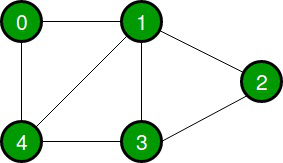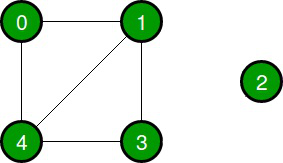GFG App
Open AppBrowser
Continue

# Java Program to Check Whether Undirected Graph is Connected Using DFS

Given an undirected graph, the task is to check if the given graph is connected or not using DFS.

A connected graph is a graph that is connected in the sense of a topological space, i.e., there is always a path from any node to any other node in the graph. A graph that is not connected is said to be disconnected.

Examples:

Input:Output: Graph is connected

Input:Output: Graph is disconnected

Approach:

1. Take a boolean visited [] array.
2. Start DFS(Depth First Search) from any of the vertexes and mark the visited vertices as True in the visited[] array.
3. After completion of DFS check if all the vertices in the visited [] array is marked as True.
4. If yes then the graph is connected, or else the graph is not connected or disconnected.

Code:

## Java

 `// Java Program to check if  ` `// an undirected graph is connected or not ` `// using DFS ` ` `  `import` `java.util.*;  ` ` `  `public` `class` `checkConnectivity { ` `     `  `    ``// Graph class ` `    ``static` `class` `Graph{ ` `         `  `        ``int` `vertices; ` `        ``// Linked list for adjacency list of a vertex ` `        ``LinkedList adjacencyList []; ` ` `  `        ``@SuppressWarnings``(``"unchecked"``) ` `        ``public` `Graph(``int` `vertices) ` `        ``{ ` `            ``this``.vertices = vertices; ` `            ``adjacencyList = ``new` `LinkedList[vertices]; ` `           `  `            ``for` `(``int` `i = ``0``; i(); ` `            ``} ` `        ``} ` `         `  `        ``// Function for adding edges ` `        ``public` `void` `addEdge(``int` `source, ``int` `dest) ` `        ``{ ` `            ``adjacencyList.addFirst(dest); ` `            ``adjacencyList[dest].addFirst(source); ` `        ``} ` `    ``} ` ` `  `    ``// Function to check if the graph is connected or not ` `    ``public` `void` `isConnected(Graph graph){ ` ` `  `        ``int` `vertices = graph.vertices; ` `        ``LinkedList adjacencyList [] = graph.adjacencyList; ` ` `  `        ``// Take a boolean visited array ` `        ``boolean``[] visited = ``new` `boolean``[vertices]; ` ` `  `        ``// Start the DFS from vertex 0 ` `        ``DFS(``0``, adjacencyList, visited); ` ` `  `        ``// Check if all the vertices are visited ` `        ``// Set connected to False if one node is unvisited ` `        ``boolean` `connected = ``true``; ` `       `  `        ``for` `(``int` `i = ``0``; i adjacencyList [], ``boolean``[] visited){ ` ` `  `        ``// Mark the vertex visited as True ` `        ``visited = ``true``; ` ` `  `        ``// Travel the adjacent neighbours ` `        ``for` `(``int` `i = ``0``; i

Output

```Graph 1:- Graph is connected
Graph 2:- Graph is disconnected
```

My Personal Notes arrow_drop_up